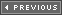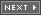### Basic Ad Hoc Collision Response

As I explained earlier in the chapter, two kinds of collisions exist: elastic and non-elastic. Elastic collisions are collisions where both kinetic energy and momentum are conserved in the colliding objects while non-elastic collisions don't conserve these values and energy is converted to heat and/or used for mechanical deformations.

Most video games don't even try to mess with non-elastic collisions and stick to simplified elastic collisions since they themselves are hard enough to compute. Before I show you the real way to do it let's use the other side of our brains. Game programmers that don't know anything about elastic or inelastic collisions have been faking collisions for years and we can do the same.

#### Simple x,y Bounce Physics

Take a look at Figure 13.20. It depicts a fairly common collision problem in games, that is, bouncing an object off the boundaries of the screen. Given the object has initial velocity (xv,yv), the object can hit any of the four sides of the screen. If one object collides with another object that has mass much greater than the colliding object, then the collision is much simplified since we only need to figure out what happened to the single object that's doing the colliding rather than two objects. A pool table is a good example of this. The balls have very small mass in comparison to the pool table.

##### Figure 13.20. The bouncing ball.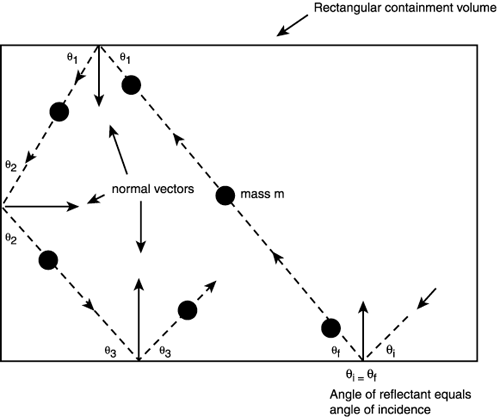When a ball hits one of the sides then it always reflects off the side at an angle equal and opposite to its initial trajectory, as shown in Figure 13.20. Thus all we need to do to bounce an object off a pool table-like environment that consists of hard edges that have large mass is to compute the normal vector direction, the direction that the object struck at, and then reflect the object at the same angle as shown in Figure 13.21.

##### Figure 13.21. Bouncing a ball off an irregular object with flat facets.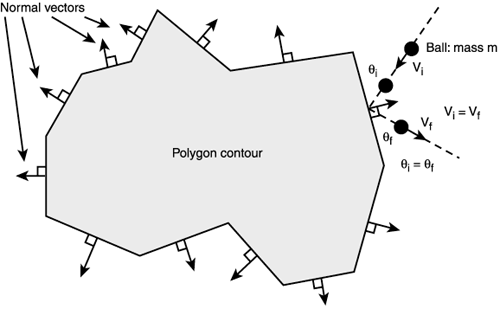Although this isn't as complex as the general elastic collision, it still takes a bit of trigonometry, so there's got to be a simpler way! And of course there is. The trick is to understand the physics model that you're trying to model. Then the idea is to see if you can solve the problem in some other way since you have exact knowledge of all the conditions. Here's the trick: Instead of thinking in terms of angles and all that, think in terms of results. The bottom line is if the object hits a wall to the east or west then you want to reverse its X velocity while leaving its Y velocity alone. And similarly on the north and south walls, you want to reverse the Y velocity and leave the X velocity alone. Here's the code:

```// given the object is at x,y with a velocity if xv,yv
// test for east and west wall collisions
if (x >= EAST_EDGE || x <= WEST_EDGE)
xv=-xv; // reverse x velocity

// now test for north and south wall collisions
if (y >= SOUTH_EDGE || y <= NORTH_EDGE)
yv=-yv; // reverse y velocity
```

And amazingly the object will bounce off the walls. Of course, this simplification only works well for horizontal and vertical barriers. You'll have to use the more general angle calculation for walls or barriers that aren't co-linear with the x and y axes.

TRICK

If you want to use the preceding technique as a quick cheat to make objects bounce off of each other, simply assume that each object is a bounding rectangle from the other object's point of view. Enact the collision and then re-compute the velocities. Figure 13.22 illustrates this.

##### Figure 13.22. A simple cheat for object-to-object collisions.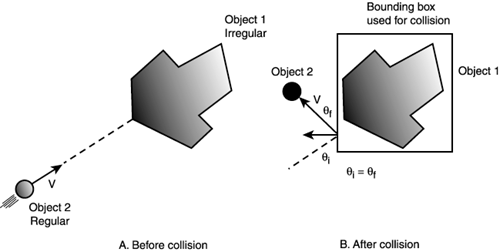As an example of using these techniques, I have created a demo named DEMO13_6.CPP|EXE (16-bit version, DEMO13_6_16B.CPP|EXE) that models a pool table with balls that never stop bouncing. Figure 13.23 shows a screen shot of the game in action. Note that when running the simulation the balls don't hit each other, just the edges of the pool table.

##### Figure 13.23. Simple collision pool table model.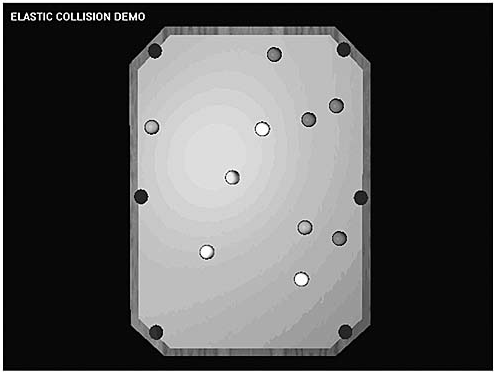#### Computing the Collision Response with Planes of Any Orientation

Using rectangles as bounding collision volumes works okay if you write pong games, but this is the 21st century, baby, and we need just a little bit more! What we need to do is derive the reflection equations for a vector reflecting off a flat plane. This is shown in part A of Figure 13.24 in 2D (the 3D case is the same). To solve the problem, first we have to make an assumption about what will happen when an object that's very small and perfectly elastic hits a wall. I think we can already come to the conclusion that it will bounce off the wall at the same angle it arrived at. Therefore, the angle of reflection (the angle of departure by the object after the collision) equals the angle of incidence (the incoming angle before the collision) relative to the normal, or perpendicular to the wall. Now, let's see the math…

##### Figure 13.24. The vector reflection problem illustrated.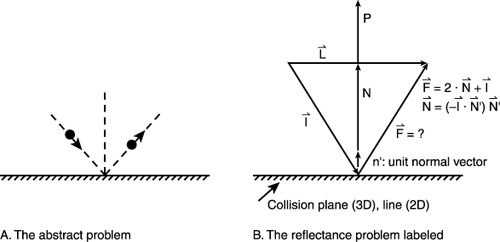Solving the problem requires nothing more than a vector geometry construction, but it's not totally trivial.

TIP

If you ever try to get a game programming job, I can almost guarantee they will ask you this question on the interview because it's deceptively complex. Luckily, you can just read this section and blurt out the answer, and they'll think you're a genius! Let's get started.

Part B of Figure 13.24 depicts the problem. Note that there isn't an x,y axis. It's unnecessary since we're using vectors and I want the problem to be totally general.

The problem can be stated like this:

Given the initial vector direction I and the normal to the plane N', find F.

Before we get crazy, let's talk about the normal vector. The normal vector N' is just the normalized version of P, but what is P? N is just the perpendicular to the plane or line that the ball is hitting that we want the ball to bounce off of. We can determine P in a number of ways; we might have pre-computed it and stored it in a data structure or we can figure it out on-the-fly.

There are a number of ways to do this depending on the representation of the "wall." If the wall is a plane in 3D then we can extract P based on the point-normal form of a plane:

```nx(x – x0) + ny(y – y0) + nz(z – zo) = 0
```

The normal vector is just P=<nx, ny, nz>. To make sure the normal is normalized or a unit vector then you divide each element by the total length—remember?

```N' = <nx, ny, nz >/|P|
```

Where |P| is the length and is computed like this:

```|P| = sqrt(nx2 + ny2 + nz2)
```

In general, the length of a vector is the square root of the sum of squares of its components.

On the other hand if your collision line is a line in 2D or a segment then you can compute the normal or perpendicular by finding any vector that is perpendicular to the line. Thus if the line is in the form of two endpoints in 2D as shown in Figure 13.25 like this:

##### Figure 13.25. Computing the perpendicular to a line.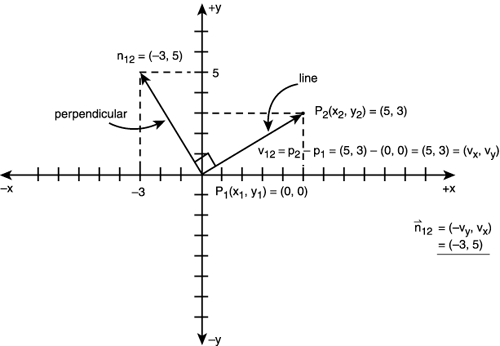Given: p1(x1,y1), p2(x2,y2)

```V12 = <x2 – x1, y2 – y1> = <vx, vy>
```

The perpendicular can be found with this trick:

```P12 = <nx, ny> = <-vy, vx>
```

The vector from p1 to p2 (remember endpoint minus initial) is

This trick is based on the definition of dot product, which states that a vector dotted with its normal equals 0, thus:

```V12 . N12 = 0
<vx, vy> . <nx, ny> = 0
```

or

```vx*nx + vy*ny = 0
```

And what makes this true is nx = -vy, ny = vx:

```vx*(-vy) + vy*(vx) = -vx*vy+vx*vy = 0
```

Nice, huh?

All right, so you know how to get the normal vector and of course you need to normalize it and make sure it has length 1.0, so N', = P/|P| which is equal to:

```N' = <-vy, vx>/sqrt((-vy)2 + vx2)
```

Now back to the derivation… At this point we have the normal vector N', which shouldn't be confused with N in the figure since N is along N', but not related to the length of P in any way. N is the projection of I along N'. Okay, that sounds like voodoo—and it is. A projection is like a shadow. If I were to shine light from the left side of the figure in the left to right direction then N would be the shadow or projection that I casts on the N' axis. This projection is the N we need. Once we have N then we can find F with a little vector geometry. First, here's N:

```N = (-I . N')*N'
```

This states that N (which is the vector projection of I on N') is equal to the dot product of –I and N' and then multiplied by the vector N'. Let's take this apart in two chunks. First the term (-I . N') is just a scalar length like 5; it's not a vector. This is a handy thing about dot products: If you want to find the shadow of one vector (projection) then you can dot it with the unit version of the vector in question, thus you can resolve the components of a vector into any direction you want. Basically, you can ask, "What's R in the V' direction?" Where V' is normalized. Therefore, the first part (-I . N') gives you a number (the –1 is just to flip the direction of I). But, you need a vector N, so all you do is multiply the number by the unit vector N' (vector multiplication) and, whammo, you have N.

Once you have N it's all bedrock, baby, just do some vector geometry and you can find F:

```L = N + I
```

and

```F = N + L
```

substituting L into F,

```F = N + (N + I)
```

Therefore,

```F = 2*N + I
```

Burn that last line into your skull. It could be the difference between Burger King and DreamWorks Interactive—right, Rich?

#### An Example of Vector Reflection

When I used to study mathematics, I used to read things like "R is a closed ring with an isomorphism on Q's kernel." I wouldn't be as nutty as I am today if they just gave me an example once in a while! Alas, I don't want you to end up running naked in the streets with a cape—one naked super hero game programmer is enough, so let's try a real example.

Figure 13.26 shows the setup of the problem. I have made the bounce plane co-linear with the x-axis to make things easier.

##### Figure 13.26. A numerical example of vector reflection.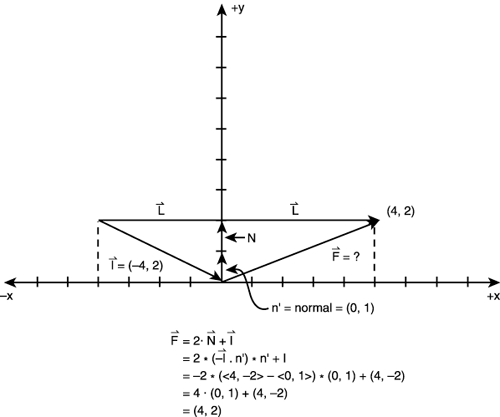The initial velocity vector of our object is I=<4,-2>, N'=<0,1>, and we want to find F. Let's plug everything into our equation:

```F =  2*N + I
=  2*(-I . N')*N' + I
= -2*(<4,-2> . <0,1>)*<0,1> + <4,-2>
= -2*(4*0 + -2*1)*<0,1> + <4,-2>
= 4*<0,1> + <4,-2>
= <0,4> + <4,-2>
= <4,2>
```

Lo and behold, if you look at Figure 13.26, that's the correct answer! Now, there's only one detail that we've left out of all this: determining when the ball or object actually hits the plane or line.

#### Intersection of Line Segments

You could probably figure this one out, but I'll give you a hand. The problem is basically a line intersection computation. But the surprise is that we are intersecting line segments, not lines; there's a difference. A line goes to infinity in both directions, while a line segment is finite, as shown in Figure 13.27.

##### Figure 13.27. Lines and line segments are very different.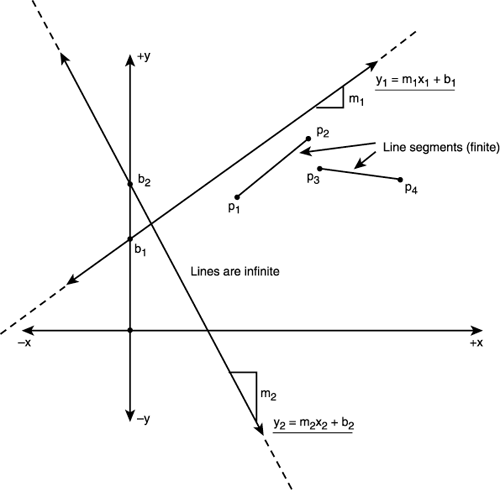The problem boils down to this: As an object moves with some velocity vector Vi, we want to detect whether the vector pierces through the collision plane or line. Why? Well, if an object is moving at velocity Vi then one frame or time click later it will be located at its current position (x0,y0)+Vi, or in terms of components:

```x1 = x0 + vix
y1 = y0 + viy
```

Therefore, you can think of the velocity vector as a line segment that leads the way to wherever the object we are drawing is going. In other words, we want to determine whether there is an intersection point (x,y) of the line segments. Here's the setup:

Object Vector Segment: S1 = <p1(x1,y1) – p0(x0,y0)>

Boundary Line Segment: S2 = <p3(x3,y3) – p2(x2,y2)>

You need the exact intersection point (x,y), so that when you compute the reflection vector F you start its initial position at (x,y). This is shown in Figure 13.28. The problem seems simple enough, but it's not as easy as you think. Intersecting two lines is as easy as solving a system of 2 equations, but determining if two line segments intersect is a little harder. That's because that although the segments are lines, they are finite, so even if the lines that the segments run along intersect, the segments may not. This is shown in Figure 13.29. Therefore, you need to not only determine where the lines that the segments define intersect, you need to see if this point is within both segments! This is the hard part.

##### Figure 13.28. Intersection and reflection.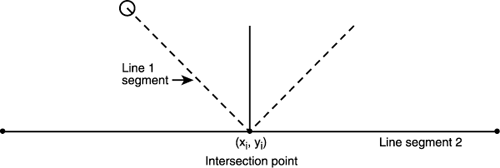##### Figure 13.29. Intersecting and non-intersecting segments.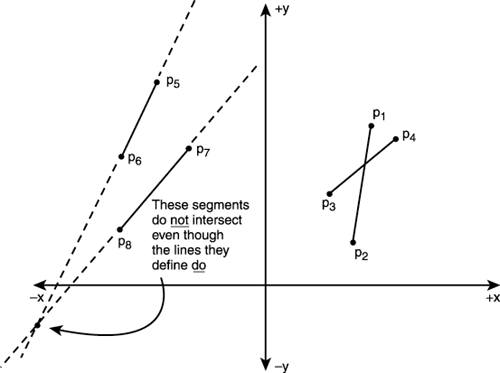The trick to solving the problem is using a parametric representation of each line segment. I'll call U the position vector of any point on S1 and V the position vector of any point on S2:

Equation 1: U= p0 + t*S1

Equation 2: V= p2 + s*S2

with the constraint that (0 <= t <= 1), (0 <= s <= 1).

Figure 13.30 shows what these two equations represent.

##### Figure 13.30. The parametric representation of U and V.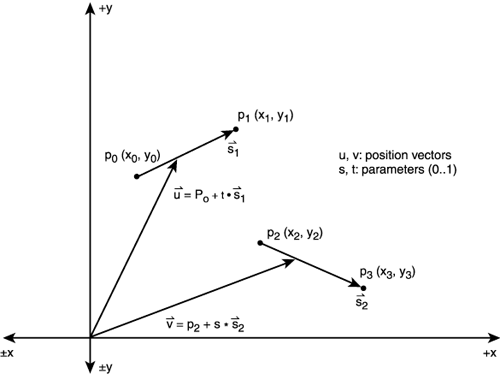Referring to the figure, we see that as t varies from 0 to 1 the line segment from p0 to p1 is traced out and similarly as s varies from 0 to 1, the line segment from p2 to p3 is traced out. Now we have all we need to solve the problem. We solve equations 1 and 2 for s,t and then plug the values back in to either equation and find the (x,y) of the intersection. Moreover, when we find (s,t) if either of them is not in the range (0 to 1) then we know that the intersection was not within the segments. Pretty neat, huh? I'm not going to every detail of the entire derivation since this is in about 100,000 math books, but I'll show you the important stuff:

Given:

```U = p0 + t*S1
V = p2 + s*S2
```

solve for (s,t) when U = V,

```p0 + t*S1 = p2 + s*S2

s*S2 – t*S1 = p0 – p2
```

Breaking the last equation into (x,y) components,

```s*S2x – t*S1x = p0x – p2x
s*S2y – t*S1y = p0y – p2y
```

We have two equations, two unknowns, push into a matrix and solve for (s,t):

```|S2x     -S1x | |s| = |(p0x – p2x)|
|S2y     -S1y | |t|   |(p0y – p2y)|
A          X  =       B
```

Using Cramer's Rule we have the following: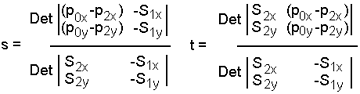MATH

Cramer's rule states that you can solve a system of equations AX=B, by computing xi = Det(Ai)/Det(A). Where Ai is the matrix formed by replacing the ith column of A with B.

MATH

The determinate (Det) of a matrix in general is rather complex, but for a 2x2 or 3x3 it is very simple to remember. Given a 2x2 matrix the determinate can be computed as follows:

```A = |a b|  Det(A) = (a*d – c*b)
|c d|
```

Multiplying all this stuff out, you get

```s = (-S1y*(p0x–p2x) + S1x*(p0y–p2y))/(-S2x*S1y + S1x*S2y)
t = ( S2x*(p0y–p2y) - S2y*(p0x–p2x))/(-S2x*S1y + S1x*S2y)
```

Then once you have found (s,t), you can plug either of them into

```U = p0 + t*S1
V = p2 + s*S2
```

and solve for U(x,y) or V(x,y). However, for s,t to be valid both of them must be in the range of (0..1). If either of them is out of range then there is NO intersection. Referring to the worked example in Figure 13.31, let's see if the math works:

##### Figure 13.31. A worked line segment intersection example.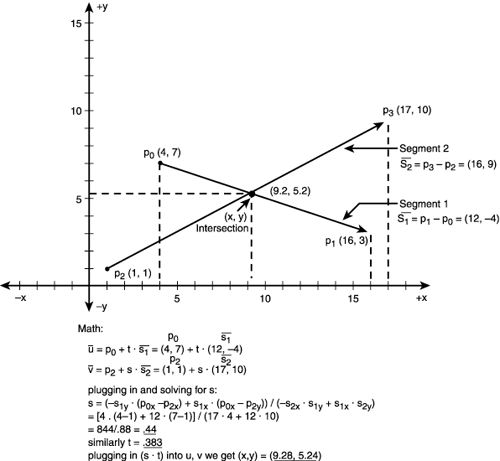TRICK

There's no need to test for intersections of two lines segments if their bounding boxes don't overlap.

```p0=(4,7), p1=(16,3),  S1=p1-p0=<12,-4>
p2=(1,1), p3=(17,10), S2=p3-p2=<16,9>
```

And we know that

```s = (-S1y*(p0x–p2x) + S1x*(p0y–p2y))/(-S2x*S1y + S1x*S2y)
t =  (S2x*(p0y–p2y) - S2y*(p0x–p2x))/(-S2x*S1y + S1x*S2y)
```

Plugging in all the values we get

```s = (4*(4-1) + 12*(7-1))/(17*4 + 12*10)   = 0.44
t = (17*(7-1) – 10*(4-1))/ (17*4 + 12*10) = 0.383
```

Since both 0<=(s,t)<=1, we know that we have a valid intersection and thus either s or t can be used to find the intersection point (x,y). Let's use t, shall we?

```U(x,y) = p0 + t*S1
= <7,7> + t*<12,-4>
```

Plugging in t=.44, we get

```= <7,7> + 0.44*<12,-4> = (9.28, 5.24)
```

which is indeed the intersection. Isn't math fun?

As for using all this technology, I have created a demo of a ball bouncing off the interior of an irregularly shaped polygonal 2D object. Take a look at DEMO13_7.CPP|EXE (16-bit version, DEMO13_7_16B.CPP|EXE). A screen shot is shown in Figure 13.32. Try editing the code and changing the shape of the polygon.

##### Figure 13.32. A bouncing ball trapped in an irregularly shaped polygon demo.Finally, you may want to try another heuristic when finding a collision trajectory vector. In the previous example we used the velocity vector as one test segment. However, it may make more sense to create a vector that is the length of the radius of the ball and then drop it from the center perpendicular to the edge being tested. This way you catch scathing collisions, but it's a bit more complex and I'll just leave it as an exercise.

 I l@ve RuBoard